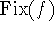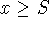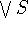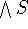## Index

REMOVE
Xia's Reduction Algorithm
k-null
Clique Graphsk-null OrderedThe Lefschetz NumberIntroductionIntroductionIntroductionIntroduction
Abian-Brown-Pelczar
Introduction, Order vs. Analysis
antichain
Isotone Relations
average case complexity
Late-breaking News ...
cancellation problem
The Cancellation Problem
chain
Introduction
chain-complete
Introduction
clique graph
Clique Graphsk-null Ordered
comparable points
Introduction
complete lattice
Introduction
conditionally complete
Order vs. Analysis
edge
Endomorphism Graphs
fence
No Title, Basic Issues, Basic Issues
fixed point
Introduction
fixed point free
Introduction
fixed point property
Introduction
graph
Endomorphism Graphs
Hammerstein integral equation
Order vs. Analysis
height
Fixed Point Theorems/Reflection Conditions
induced subgraph
Endomorphism Graphs
infimum
Introduction
lattice
Introduction
lower bound
Introduction
open questions
Open Questions
order-preserving map
Introduction
ordered set
Introduction
ordered subset
Introduction
supremum
Introduction
truncated noncomplemented lattice
Truncated Lattices
non-topological proof;tex2html_html_special_mark_quot;of fixed point theorem
More Late-Breaking News ...
upper bound
Introduction
vertex
Endomorphism Graphs
width 2
Isotone Relations
Xia's algorithm
Basic Issues
use outside fixed point theory
Late-breaking News ...

Bernd.S.W.Schroder